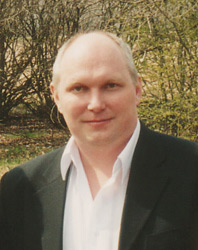Unipolar Generator with Plasma Disk
By A.V. Frolov

The unipolar generator uses Lorentz force to separate positive and negative charges in rotating   metal disk. The formula is known:

F= qVH,

where  q is charge, V is Velocity and H is intensity of magnetic field.

The approach of N-machine research groups to question of   increasing of power is conventional: to increase the velocity V or intensity H. For example, Dr. Inomata, Japan, uses magnets with superconducting coils to increase the strength of the magnetic field through the disk. But it is not the optimal way. The density of free electrons in a copper disk is the same in this case.

It is possible to use another approach. Total force (and  power in load) is proportional to the density ot rotating plasma:

SF=NQVH

where N is the number of particles moving in field H.
In some cold fusion systems this density of protons in cathode is about 10 29 (m-1) that correspond to enormous N in above formula. The utilization of such type of cathode that is saturated by protons in scheme of unipolar generator as rotating disk allow to create new power possibilities for such sort free energy systems.

Note that in this case we will work with rotating plasma and this technology is similar to the idea of magnetohydrodynamical generator.